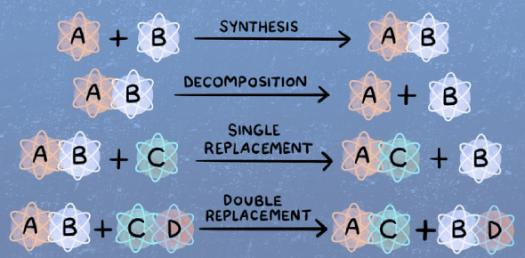# Chemistry: Chemical Reaction And Equation Questions!

15 Questions | Attempts: 19323
ShareSettingsThere are two changes that can be observed when we mix substances together and this is either physical or chemical changes. A chemical reaction involves the rearrangement of the molecular structure of a substance. Test out your understanding of chemical reactions by taking up the quiz below. All the best and come back for more quizzes like these to aid in your revision.

• 1.
What are the coefficients of the correctly balanced equations? ----Fe2O3    +  ----CO  ----Fe    +    ---CO2
• A.

0,2,2,3

• B.

1,3,2,3

• C.

1,2,2,2

• D.

2,6,4,3

• 2.
CaO- calcium Oxide reaction with water is regarded as exothermic. A student mixes these two products in a test tube and touches its side surface. Which of the following statement correctly describes the student’s observation?
• A.

The test tube becomes cold due to release of heat energy

• B.

The test tube becomes hot due to release of heat energy

• C.

The test tube becomes hot due to absorption of heat energy

• D.

The test tube becomes cold due to absorption of heat energy

• 3.
Which one is a double displacement reaction?
• A.

BaCl2 + Na2SO --------> BaSO4 + 2NaCl

• B.

CaO + H2O ------------> Ca(OH)2

• C.

2Pb(NO3)2          --------> 2PbO + 4NO2 + O2

• D.

Zn + 2AgNO3      ----------> Zn(NO3)2 + 2Ag

• 4.
Which of the following are exothermic processes-
1. Reaction of water with quick lime
2. Dilution of an acid
3. Evaporation of water
4. Sublimation of camphor crystals
• A.

(i) and (ii)

• B.

(ii) and (iii)

• C.

(i) and (iv)

• D.

(iii) and (iv)

• 5.
Zinc + Sulphuric acid → Zinc sulphate + (X) What will be the product “X”?
• A.

Hydrogen

• B.

ZnO

• C.

Zinc Sulphite

• D.

Nitrogen

• 6.
Which of the statements about the reaction below are incorrect?     2PbO(s) + C(s) →      2Pb(s) + CO2 (g)     i. Lead is getting reduced.     ii. Carbon dioxide is getting oxidized.    iii. Carbon is getting oxidized.    iv. Lead oxide is getting reduced.
• A.

(i) and (ii)

• B.

(i) and (iii)

• C.

(i), (ii) and (iii)

• D.

(iv) only

• 7.
Which of the following is not a balanced equation?
• A.

H2 + O2                     H2O

• B.

Mg + CuSO2         MgSO4 + Cu

• C.

NaOH + HCl       NaCI + H2O

• D.

Zn + S                 ZnS

• 8.
Aluminum reacts with copper sulphate to produce
• A.

Cu and Al2(SO4)3

• B.

CuO and Al2(SO4)3

• C.

Cu, Al2S3 and SO2

• D.

CuO, Al2S3 and SO2

• 9.
What happens when dilute HCl is added to iron filings? Tick the correct answer.
• A.

Hydrogen gas and iron chloride are produced.

• B.

Chlorine gas and iron hydroxide are produced.

• C.

No reaction takes place.

• D.

Iron salt and water are produced.

• 10.
Solid calcium oxide reacts vigorously with water to form calcium hydroxide accompanied by liberation of heat. This process is called slaking of lime. Calcium hydroxide dissolves in water to form its solution called lime water. Which among the following is (are) true about slaking of lime and the solution formed ?   i. It is an endothermic reaction   ii. It is an exothermic reaction   iii. The pH of the resulting solution will be more than seven   iv. The pH of the resulting solution will be less than seven
• A.

(i) and (ii)

• B.

(ii) and (iii)

• C.

(i) and (iv)

• D.

(iii) and (iv)

• 11.
Which among the following are endothermic processes? i. Reaction of quick lime with water ii. Dilution acid with water iii. Evaporation of water iv. Sublimation of solid ammonium chloride
• A.

(i) and (ii)

• B.

(ii) and (iii)

• C.

(i) and (iv)

• D.

(iii) and (iv)

• 12.
Barium chloride on reacting with ammonium sulphate forms barium sulphate and ammonium choloride. Which of the following correctly represents the type of the reaction involve? i. Displacement reaction ii. Precipitation reaction iii. Combination reaction iv. Double displacement reaction
• A.

(i) , (iii)

• B.

(ii), (iii)

• C.

(iii)

• D.

(ii), (iv)

• 13.
A dilute ferrous sulpahte solution was gradually added to the beaker containing acidified KMnO4 solution. The light purple colour of the solution fades and finally disappears. Which of the following is the correct explanation for the observation?
• A.

KMnO4 is oxidizing agent, it oxidizes FeSO4

• B.

FeSO4 acts as an oxidizing agent and oxidizes KMnO4

• C.

The color disappears due to dilution: no reaction is involved

• D.

KMnO4 is an unstable compound and decomposes in presence of FeSO4 to a colorless compound.

• 14.
Which of the following reactions is used in which washing of walls?
• A.

2Ca+O2                     2CaO

• B.

Ca(OH)2  heat          CaO+ H2O

• C.

Ca(OH)+CO2     CaCO3+H2O

• D.

CaO+H2O          Ca(OH)2

• 15.
The electrolytic decomposition of water gives H2 and O2 in the ration of
• A.

1:2 by volume

• B.

2:1 by volume

• C.

8:1 by mass

• D.

1:2 by mass

## Related TopicsBack to top
×

Wait!
Here's an interesting quiz for you.